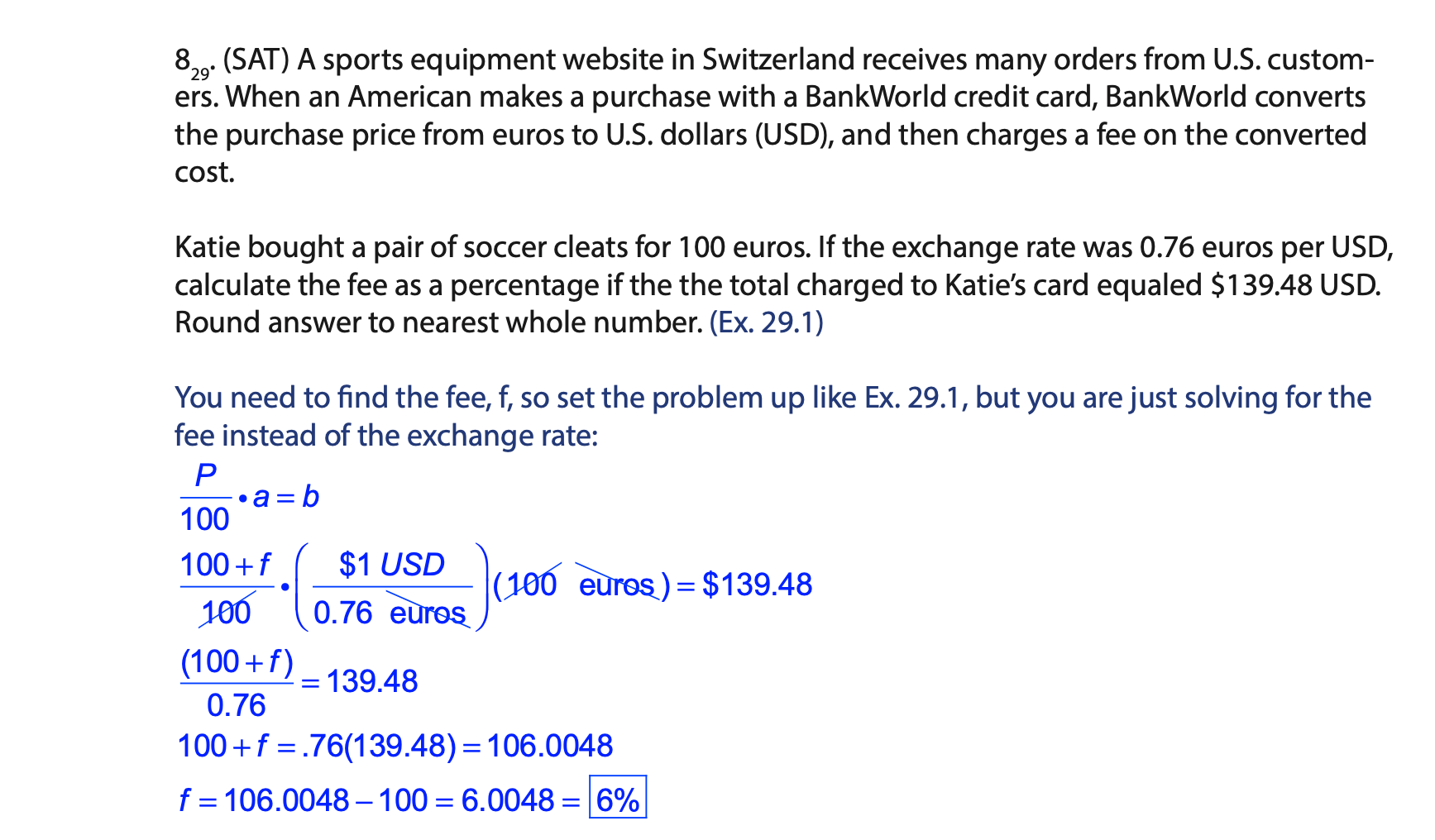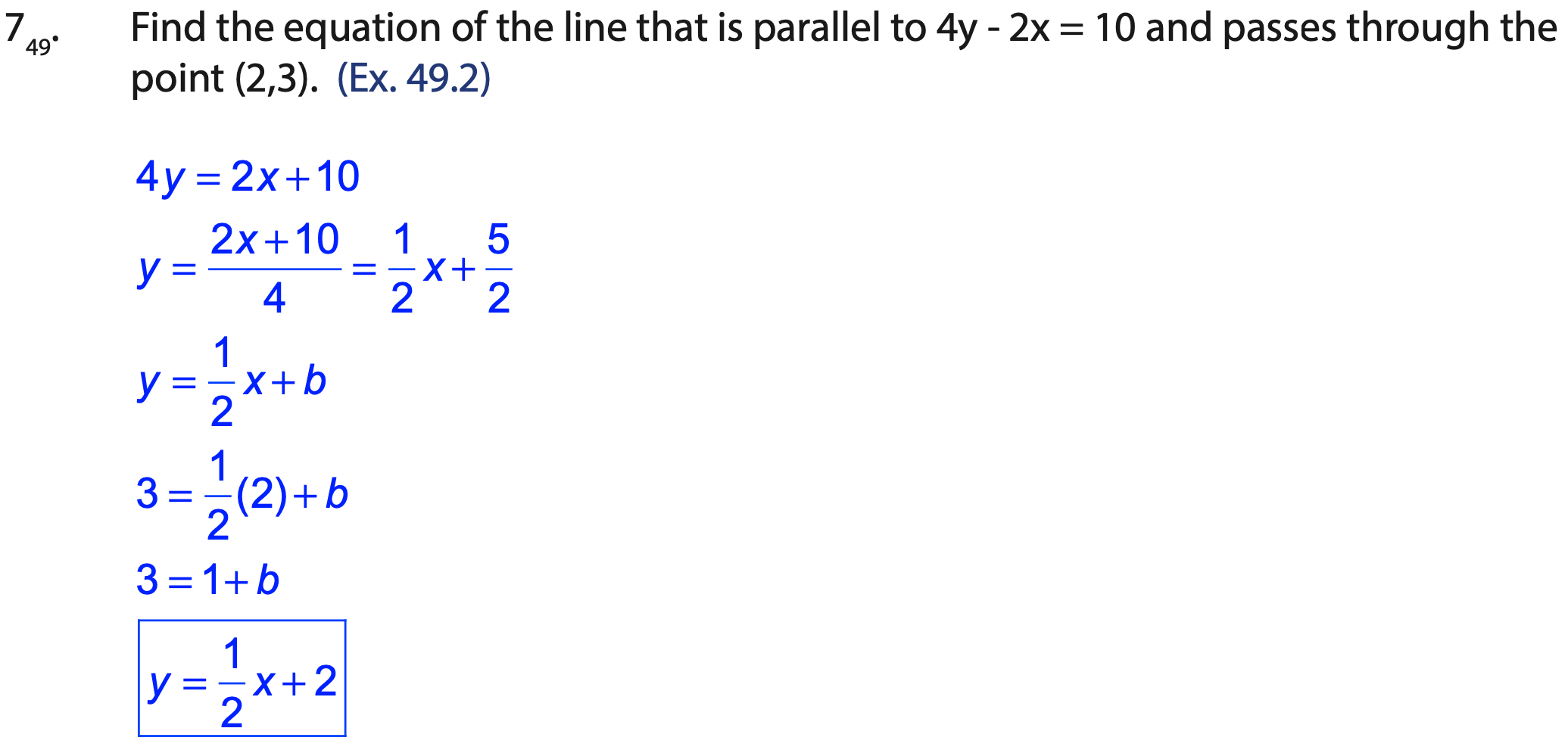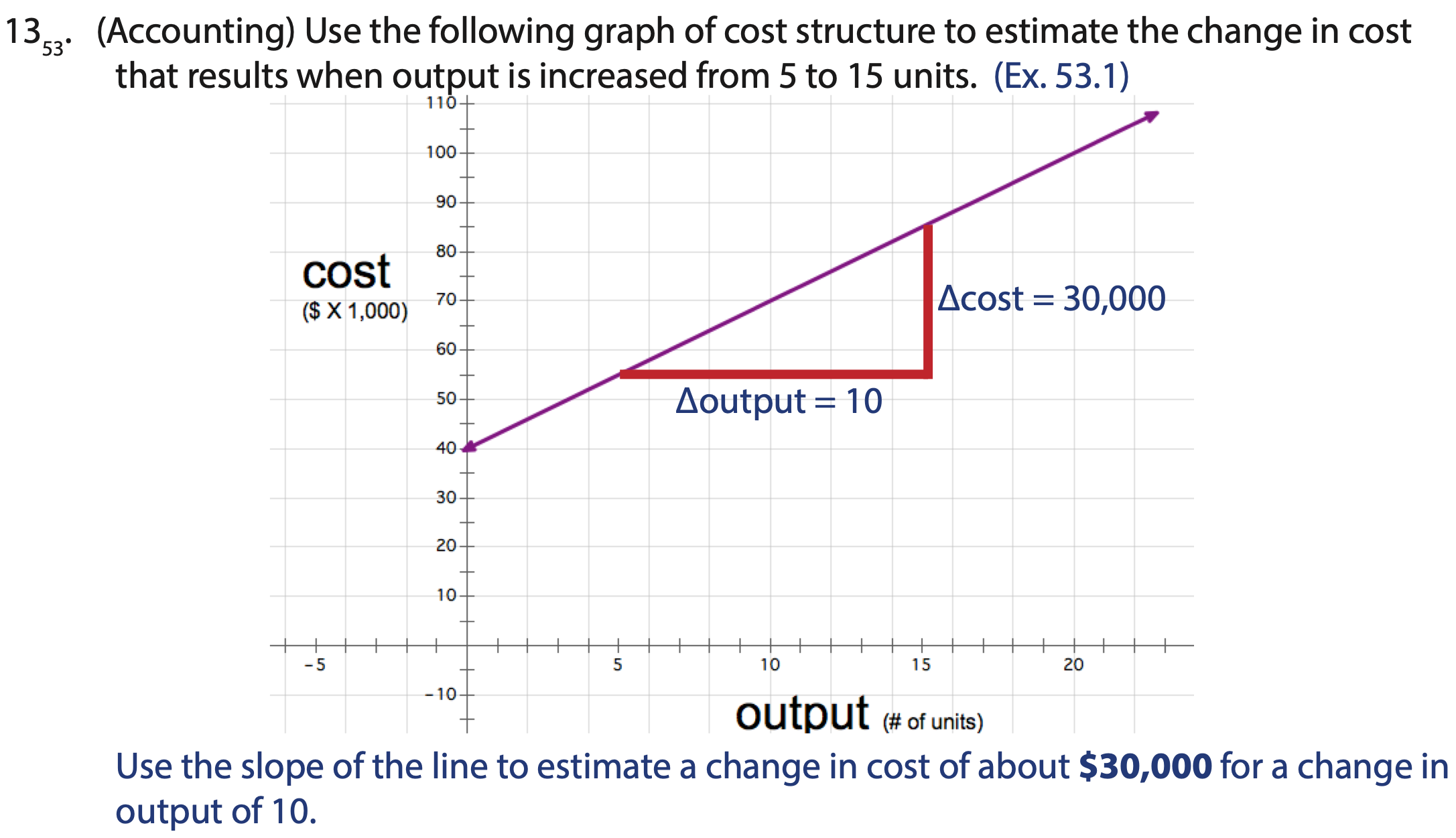# Samples for Shormann Algebra 1 with Integrated Geometry

This page contains samples of the video lessons, eTextbook, eSolutions Manual, and the video solutions for several lessons.

1. How Does the Course Work?

2. How to Monitor Student Progress

LESSON 1 Samples

Video Lecture for Lesson 1

Textbook Pages with Practice Sets: Lesson 1

Lesson 30 Samples

Video Lecture for Lesson 30

Textbook Pages with Practice Sets  Lesson 30

Sample of the Video Solution for #8 in Lesson 30

Sample of the PDF Solution (#8 in Lesson 30)Lesson 51 Samples

Video Lecture for Lesson 51

Textbook Pages for Lesson 51

Sample Video Solution for Lesson 51

Sample PDF Solution for #7 in Lesson 51Lesson 76 Samples

Video Lecture for Lesson 76

Textbook Pages with Practice Set for Lesson 76

Sample Video Solution for Lesson 76

Sample PDF Solution for Lesson 76